### Grab This Third Grade Math Multiply With 1 Color By Number and Task Cards Resource Bundle for Your Class Today!

Finding class time during the day to allow students to work on their basic multiplication facts can be challenging! But knowing those facts quickly will help your students master the harder math skills ahead of them! You will love the no prep, print and go ease of this 3rd Grade Math Multiply With 1 Color By Number and Task Cards Resource includes 6 pages for introducing or reviewing multiplication by focusing only on Multiply By 1.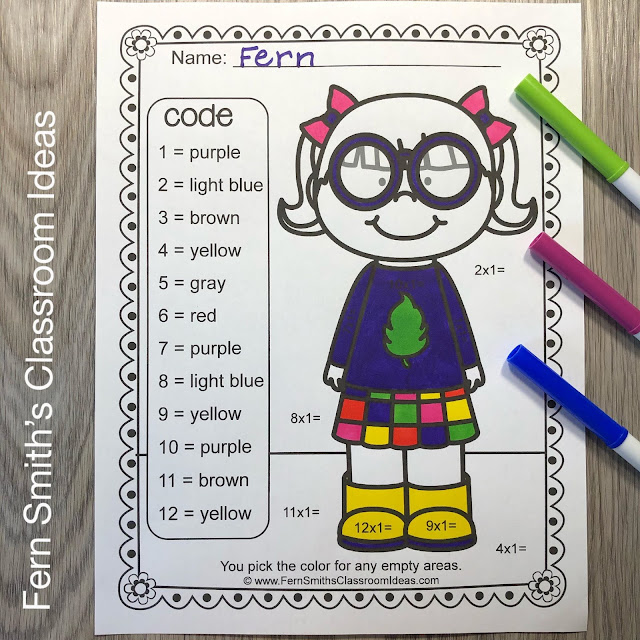You will love the no prep, print and go ease of these six Multiply With 1 Color By Number printables. This 3rd Grade Math Multiply With 1 Color By Number and Task Cards Bundle also includes 24 Multiplication Task Cards, 2 Different Styles of Recording Worksheets, with two types of directions, 1 with the directions for the students to show their work with a quick draw, and 1 with the directions for the students to write their answers only. Multiplication is an essential skill to master in third grade. Students need to be able to interpret products of whole numbers. In addition to being able to fluently multiply within 100.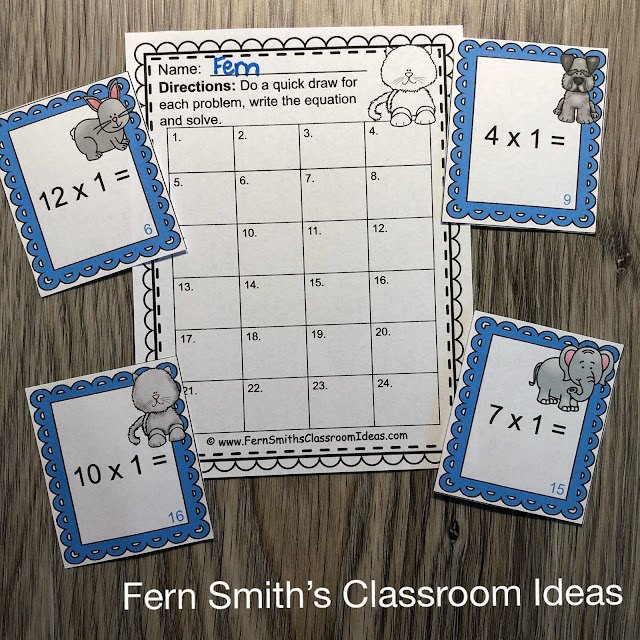Grab This Third Grade Math Multiply With 1 Color By Number and Task Cards Resource Bundle for Your Class Today!
Each Color By Number page in this resource reinforces those skills by only focusing on multiplying by 1. Your students will adore their finished pages with the cute children and animal themes in these Color By Number Worksheets while learning and reviewing important skills at the same time! My students LOVED Task Cards and your students will too! You can dedicate one of your math centers, math workstations, as a task card center. By changing out the skill each week, Multiply with 1, Multiply with 2, and so on, your students already know the directions for using the task cards.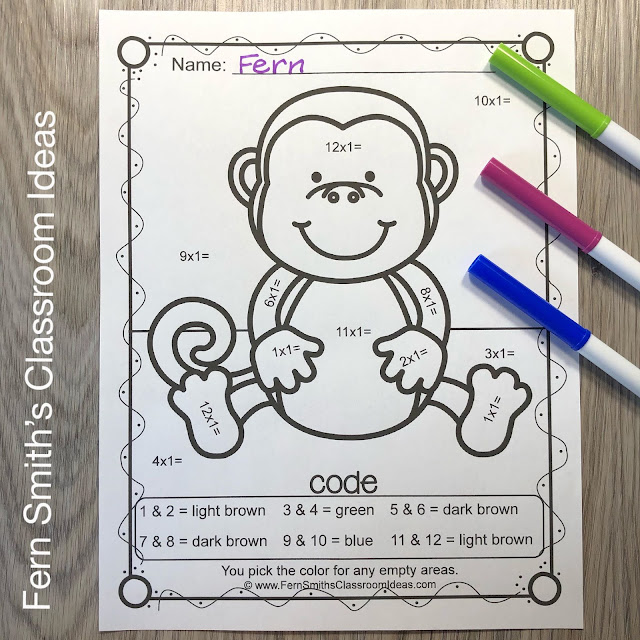Grab This Third Grade Math Multiply With 1 Color By Number and Task Cards Resource Bundle for Your Class Today!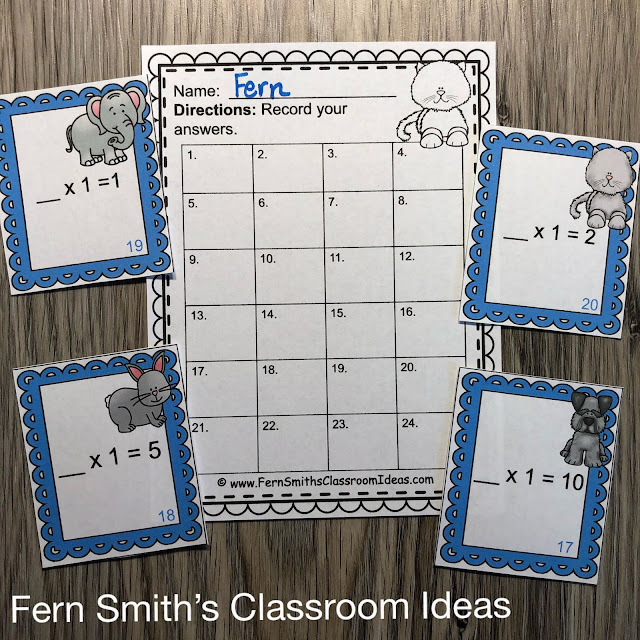Grab This Third Grade Math Multiply With 1 Color By Number and Task Cards Resource Bundle for Your Class Today!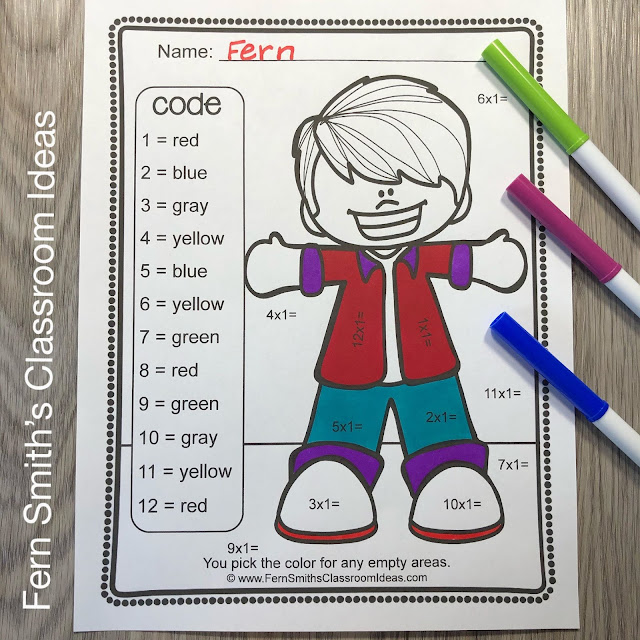Grab This Third Grade Math Multiply With 1 Color By Number and Task Cards Resource Bundle for Your Class Today!
I have no math problems on the cute little children's skin and hair, allowing your students to select any skin tone color and any hair color. The directions read, "You pick the color for any empty areas." This also allows for some creativity after their math is done by selecting additional colors of their choice for the borders and other blank remaining areas. This feature is a real hit with my teacher buyers!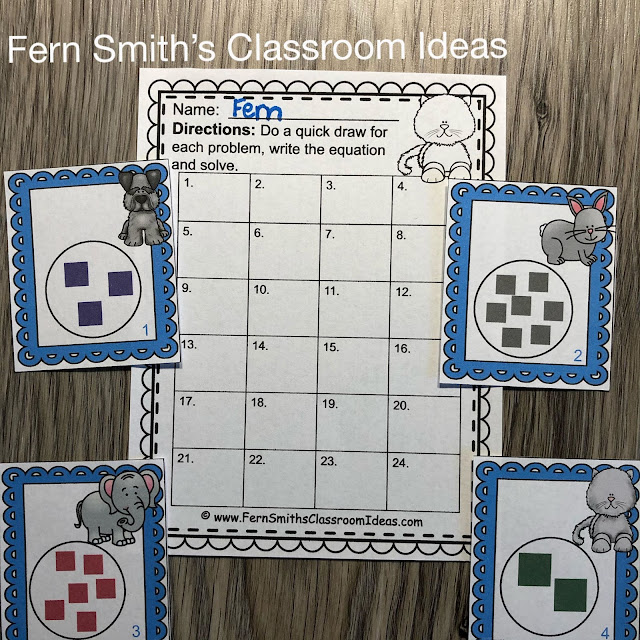Grab This Third Grade Math Multiply With 1 Color By Number and Task Cards Resource Bundle for Your Class Today!

These 3rd Grade Math Multiply With 1 Color By Number and Task Cards Resource includes: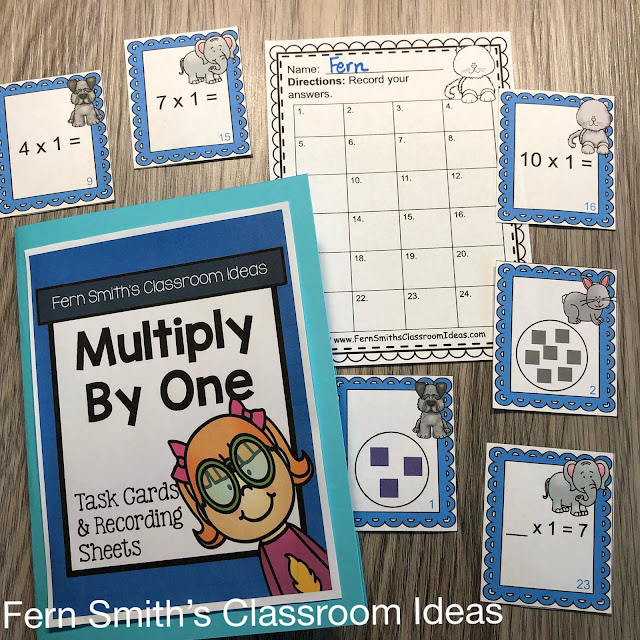Grab This Third Grade Math Multiply With 1 Color By Number and Task Cards Resource Bundle for Your Class Today!

These 3rd Grade Math Multiply With 1 Color By Number and Task Cards Resource includes:

* Printed Lesson Plan Ideas to Use this 3rd Grade Math Multiply With 1 Bundle

→ 24 Multiply With 1 Task Cards in color
→ 2 Different Recording Worksheets
→ One with the directions for the students to show their work with a quick draw.
→ One with the directions for the students to write their answers only.
→ 1 Answer Key Sheet that can also be used as a Student Self-Checking Sheet
→ 1 Multiply With 1 Task Card Folder Cover or Center Sign

* This Multiply With 1 Color By Number math resource includes:
* Three Multiply With 1 Color By Number Worksheets

Page One - A cute little girl ready for school with all multiply with 1 math problems.

Page Two - A cute little boy ready for school with all multiply with 1 math problems.

Page Three - A cute little monkey with all multiply with 1 math problems.

* Three Multiply With 1 Math Problems Worksheets

Page Four - 24 multiply with 1 math problems. These problems are in order starting with 1x1= and ending with 12x1=. The problems are repeated twice. If a full page of 24 problems is too much for your class, or a student, the page is designed to be cut in half easily for the student to only have the first twelve problems of multiplying by one. Then later they can have another practice page of just 12 problems again. {Folding the page in half is also a wonderful way to use this page.}

Page Five - 24 multiply with 1 math problems. These problems are in mixed order. The problems on this page are also repeated twice. If a full page of 24 problems is too much for your class, or a student, the page is designed to be cut in half easily for the student to only have the first twelve problems of multiplying by one. Then later they can have another practice page of just 12 problems again. {Folding the page in half is also a wonderful way to use this page.}

Page Six - 24 MISSING NUMBER math problems for multiplying with 1. The first 12 problems are in order, ___x1=1 and ending with ___x1=12. The next 12 problems are in mixed order, still starting with the missing number, for example. ___x1=3.

Pages Seven, Eight, and Nine - The answer keys that are color coded for the color by number boy, girl and animal pages.

Pages Ten, Eleven, and Twelve - The answer keys for the math problem pages, perfect for assessments, independent student reviews or substitute teacher days.

The 3rd Grade Math Multiply With 1 Task Cards also include the answer keys, which you can laminate and leave at the center to allow it to be student led, self-checking sheets. In small group lessons you can give each student three to four cards and they can help each other and review Multiply With 1 Identity Property of Multiplication by checking the answers that their partner or neighbor answered, keeping everyone involved! Keep your students engaged, alert and on their toes with this one little, cute Multiply With 1 Task Cards Resource!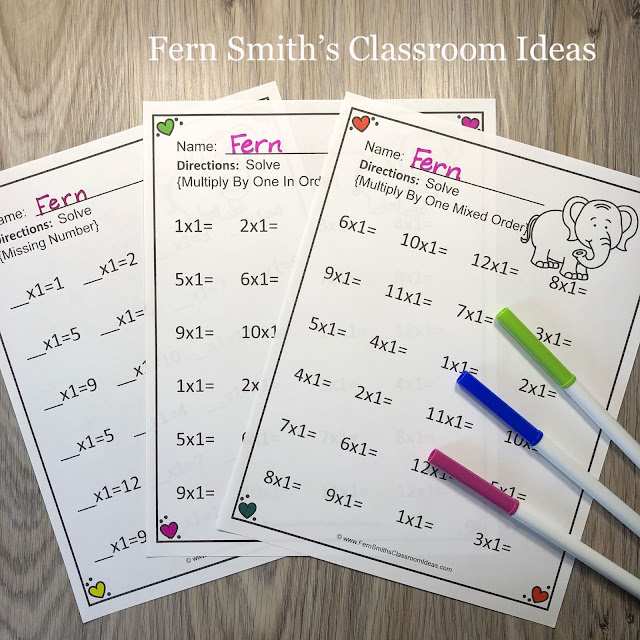Grab This Third Grade Math Multiply With 1 Color By Number and Task Cards Resource Bundle for Your Class Today!

Lesson Plan Ideas

Entire Week - Have the Task Cards at your math center, or place them in a pencil box or storage box to be a traveling math center that your students can take to their table, a rug area, or even UNDER a large classroom table for a fun, easy and free quiet work area.

Monday - Use page 5, 24 mixed order math problems, as a pre-assessment.

Tuesday - Use page 1, the cute little girl color by number page during math seat work, math centers or small group time.

Wednesday - Use page 4, 24 math problems in order, as a whole class activity. Then use page 2, the cute little boy color by number page during math seat work, math centers or small group time.

Thursday - Use page 6, 24 missing number math problems, as a way to take Multiply With 1 to the next level, with a harder concept of "what's missing?" as a whole class activity. Then use page 3, the cute little monkey color by number page during as a continued review for your math seat work, math centers, small group time, or homework. Use page 4, 24 math problems in order, as a homework review. For a change of pace, you can send page 3, the cute little animal page as homework instead.

Friday - Use page 5 again, the 24 mixed order math problems, as a final assessment.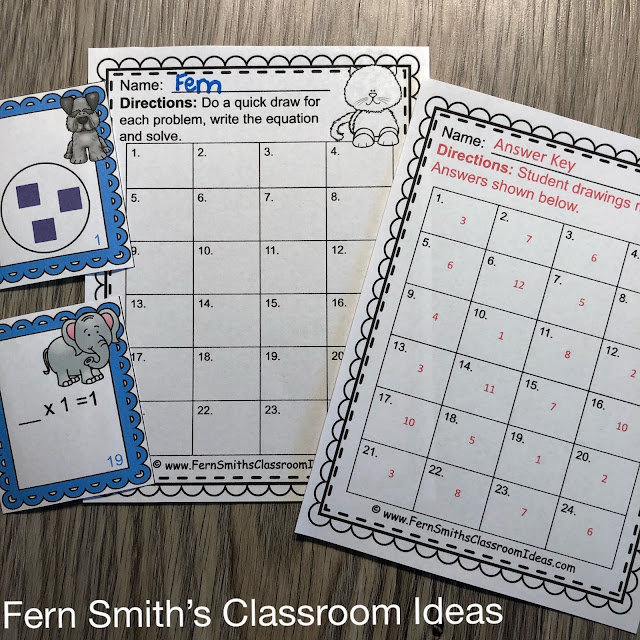Grab This Third Grade Math Multiply With 1 Color By Number and Task Cards Resource Bundle for Your Class Today!

This 3rd Grade Math Multiply With 1 Color By Number and Task Cards Resource is Part of These LARGER Bundles: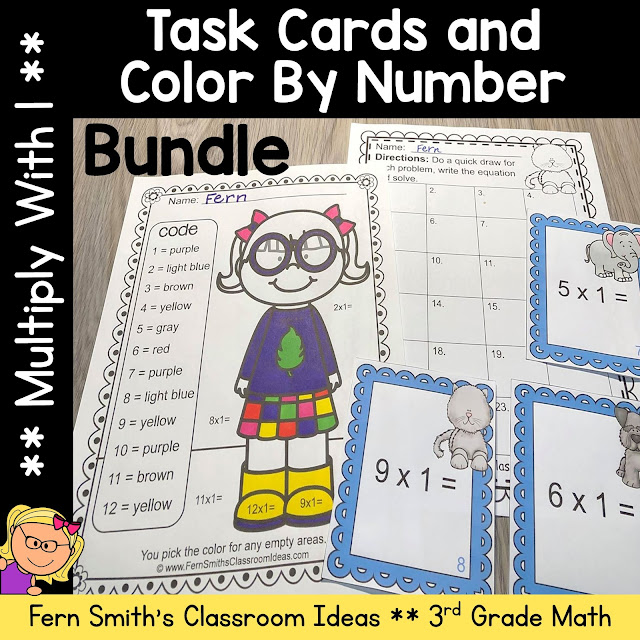Grab This Third Grade Math Multiply With 1 Color By Number and Task Cards Resource Bundle for Your Class Today!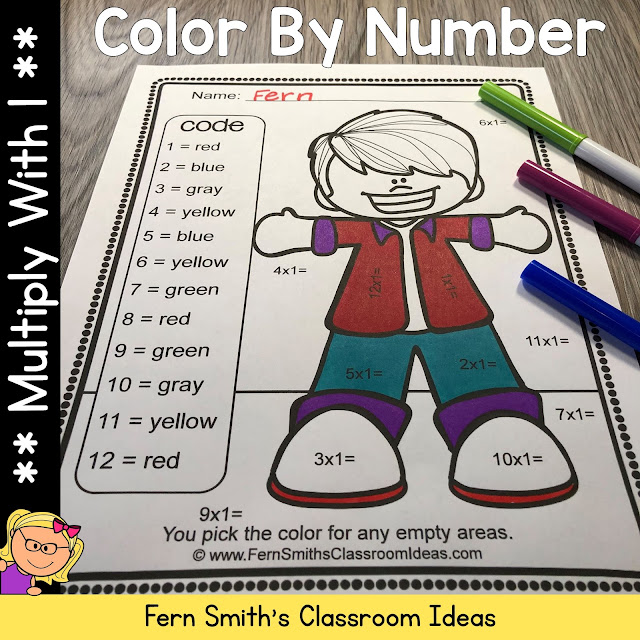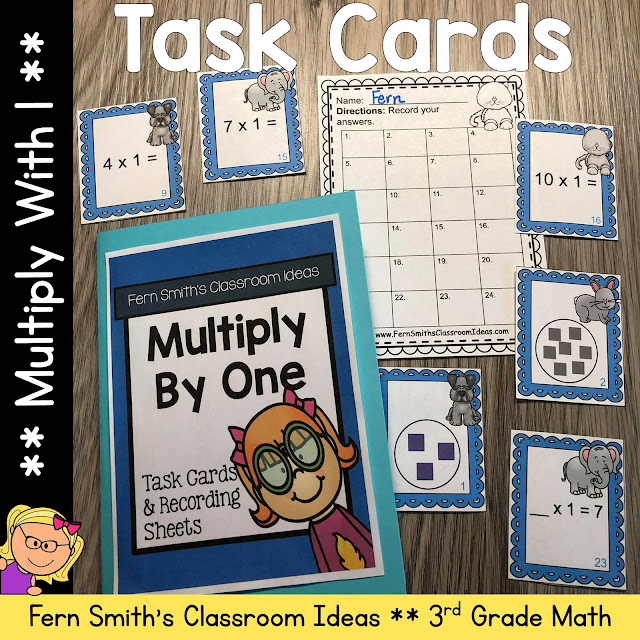Common Core State Standards
CCSS.MATH.CONTENT.3.OA.A.1
Interpret products of whole numbers, e.g., interpret 5 × 7 as the total number of objects in 5 groups of 7 objects each. For example, describe a context in which a total number of objects can be expressed as 5 × 7.

CCSS.MATH.CONTENT.3.OA.A.3
Use multiplication and division within 100 to solve word problems in situations involving equal groups, arrays, and measurement quantities, e.g., by using drawings and equations with a symbol for the unknown number to represent the problem.

CCSS.MATH.CONTENT.3.OA.B.5
Apply properties of operations as strategies to multiply and divide. Examples: If 6 × 4 = 24 is known, then 4 × 6 = 24 is also known. (Commutative property of multiplication.) 3 × 5 × 2 can be found by 3 × 5 = 15, then 15 × 2 = 30, or by 5 × 2 = 10, then 3 × 10 = 30. (Associative property of multiplication.) Knowing that 8 × 5 = 40 and 8 × 2 = 16, one can find 8 × 7 as 8 × (5 + 2) = (8 × 5) + (8 × 2) = 40 + 16 = 56. (Distributive property.)

CCSS.MATH.CONTENT.3.OA.B.6
Understand division as an unknown-factor problem. For example, find 32 ÷ 8 by finding the number that makes 32 when multiplied by 8.

CCSS.MATH.CONTENT.3.OA.B.7
Fluently multiply and divide within 100, using strategies such as the relationship between multiplication and division (e.g., knowing that 8 × 5 = 40, one knows 40 ÷ 5 = 8) or properties of operations. By the end of Grade 3, know from memory all products of two one-digit numbers.

***Don’t forget that all my new products on TpT are 40% off for 48 hours.***
Click this LINK to follow my shop & you'll be notified of these huge savings.
See you tomorrow,